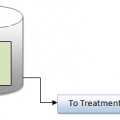## Air Blower Capacity Selection for Aeration Tank

Did you Select, correct Capacity Air blower for your aeration Tank/Basin ? Any water and wastewater treatment process Air Requirement is inevitable for clear process. many engineer is is still confusing and trouble to choose exact capacity for that application,

for your easy understand we given simple solution with example.

HP of the Blower / Fan = ( CFM X PSI ) / ( 229 X Efficiency  of the blower )

Stands for CFM : Cubic Feet per Minute

PSI : Pounds per Square Inch

for Example .. after you determine air quantity of the Aeration Basin,

here we take roughly Require Air quantity =  650 M³/Hr ; Aeration Tank Depth is 4 Metre

Now Apply that figure into above formula,

Firstly, we convert M3/Hr to CFM

1 Metre³ = 35.31 Feet³

So, 650 Metre³ air require per Hour, hence 650 /60 = 10.833 M³ require per Minute

10.83 x 35.31 = 382.41 CFM air require / Minute

Secondly, we determine PSI using Depth 1 Metre Depth Water Column builds 1.42 psi

hence 4 metre Depth created 5.68 psi =  0.4 kg /cm²

Add 1 More metre, for Liquid Depth 4 metre vertical and One or half metre free drop of pipe and distribution pipes, valve’s throttle back pressure which you have fitted in the distribution line

hence we might add 1 to 2 metre roughly that will be better.

4 + 2 = 6 metre = 8.53 psi = 0.6 Kg/cm² is OK

similarly add 10 % Air volume = 383 + 38.3 =421.3 say 425 CFM

So, apply  this figure

HP =( 425 X 8.53 ) /( 229 X 0.69) = 10.9 HP say 11 HP Power, Air Blower Capacity is OK

60 TO 78 % Efficiency have Twin Lobe Air Blower,  here we took 69% efficiency

Check Blower Efficiency from Name Plate OR straight away ask to supplier.

Now, we should find Motor Capacity in HP

Simply ,

Motor HP = Blower Capacity in HP / Motor Efficiency

Normally it range 75 to 92 %, Here we take 85%

So,   = 11/0.85 = 13 HP

we make take 15 HP in motor Side else in neck to neck 12.5 HP.## Author:T.P.Sivanandan

#### Why Fine Bubbles are Better than Coarse bubble in Aeration Tank#### How to Calculate Lime Dosage Requirement in Sedimentation Process## 7 thoughts on “Air Blower Capacity Selection for Aeration Tank”

1.S.K.Deshpande says:

HP =( 425 X 8.53 ) /( 229 X 0.69) = 10.9 HP say 11 HP Power Please recheck calculation.

1.ajay singh says:

sir from where 229 has been taken

2.T.P.Sivanandan says:

Thanks, it comes 23 HP

2.ajay k says:

Hii sir,

Can you please clarify me, what is that 229 ?
Which conversion it is representing ?

1.T.P.Sivanandan says:

It is calculation Constant

3.Sudhir kumar says:

229 is calculation contact.
Plz decribe it by formula.

4.Ben says:

How would you do the reverse and size the blower for an aerated grit chamber?
If I know the channel length, width, number of drops etc.
This would be presuming the blower was at the tank, no long run to consider, neglect atmosphere, pipe friction and all the small negligible items.
this would provide CFM and pressure at the entry of the manifold for the aeration.